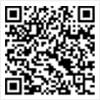# 2020MBA管综数学练习（三）

MBA报名、考试、查分时间 免费短信提醒看不清楚，换张图片

【摘要】管理类联考数学复习必须要掌握一定的方法技巧，针对不同类型题目采纳不同对策，下面环球网校考研MBA小编为大家分享2020MBA管综数学练习题，轻松搞定联考数学，希望对各位读者有帮助。

2020MBA管综数学练习(三)

1、已知f(xy)=f(x) f(y)且f′(1)=a,x≠0，求f′(x)=? (答案为a/x)

【思路1】原方程两边对Y进行求偏导

xf′(xy)=f′(y) 其中f′(xy)与f′(y)都是对y偏导数

xf′(x*1)=f′(1)=a 得 f′(x)=a/x

【思路2】当⊿x→0时，令x ⊿x=xz则z=(1 ⊿x/x)

={f[x(1 ⊿x/x)]-f(x)}/⊿x

=[f(x) f(1 ⊿x/x)-f(x)]/⊿x

=f(1 ⊿x/x)/⊿x =f′(1)/x=a/x

2、已知函数f(x y,x-y)=x2-y2, 则f对x的偏导数加f对y的偏导数等于? (a)2x-2y (b)x y

【思路1】设U=x y,v=x-y

f(u,v)=uv

f′x=f′u*u′x f′v*v′x=v*1 u*1=u v

f′y=f′u*u′y f′v*v′y=v-u

f′x f′y=u v v-u=2v=2(x-y)=2x-2y 选A

【思路2】由已知f(x y,x-y)=(x y)(x-y),

3、已知方程7x2-(k 13)x k2-k-2=0的两个实根分别在区间(0，1)和(1，2)内，则k的取值范围是什么?答案为(-2，-1)U(3，4)

【思路】画图可得f(0)>0,f(1)<0，f(2)>0代入计算即可。

4、A,B是一次随机实验的两个事件，则___

A. A-(B-A)=A-B B. A-(B-A)=A

【思路】B,利用定义可得。

5、已知随机变量X的密度的函数是：f(x)=

A、与a无关，随着m的增大而增大

B、与m无关，随着a的增大而增大

C、与a无关，随着m的增大而减少

D、与m无关，随着a的增大而减少

【思路】P{m0)= dx=Ae-m=1 A=em ,P{m= =Ae-m [1-e-a]= 1-e-a a>0 答案为B。

|

## MBA最新文章推荐MBA各地入口iPhone版